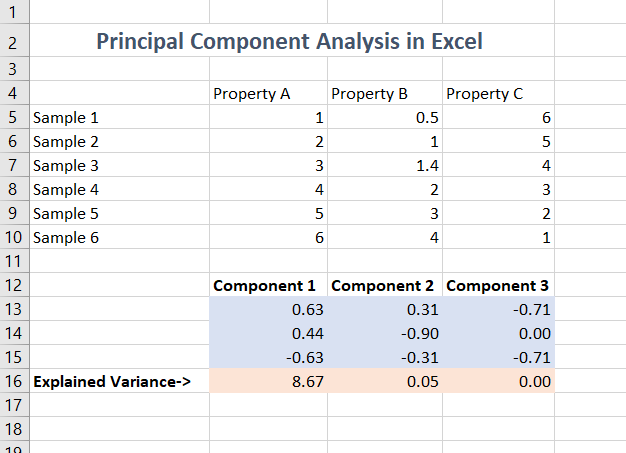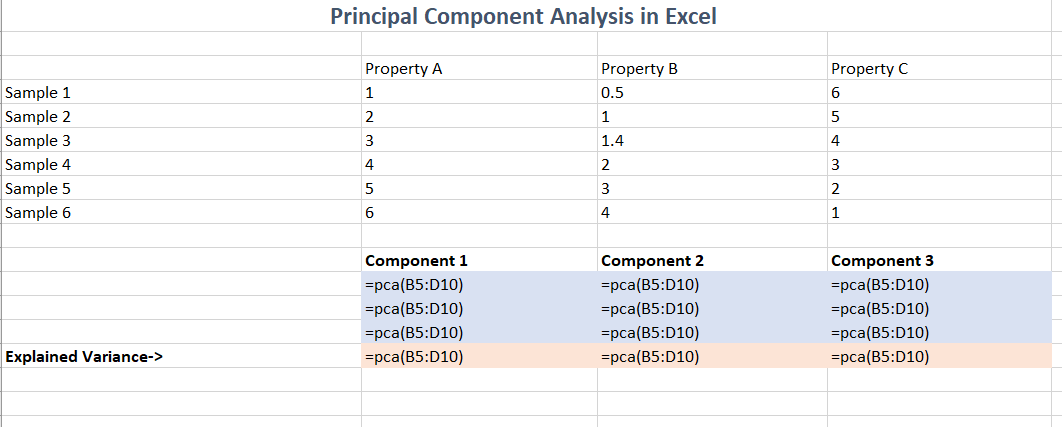If you like to fit straight lines to two dimensional datasets, you’ll probably like doing Principal Component Analysis (PCA) to multidimensional ones. It can be seen as a generalisation specifically of orthogonal distance regression to higher dimensions. There is a nice presentation in the booklet Dimension Reduction: A Guided Tour, by Burges. A nice further discussion is also presented at this blog.

In many cases it is useful to keep a keen eye on the actual data used in PCA, and in this case Excel is a great tool. It is then convenient to the PCA from within which fortunately can be done extremely easily with `python`, the `scikit-learn` python package and `xlwings` to connect excel to python.

# Setup

If you already have Python and/or xlwings installed you can skip the following two sub-sections.

## Installing python

I install the plain Windows installer, which you can get straight from python.org :

https://www.python.org/ftp/python/3.9.5

Select the option to add Python environment variables.

## Installing xlwings

There are comprehensive instructions on the xlwings website for the package and specifically for the addin. In brief, it consists of:

Installing the xlwings package via pip:

``````pip install xlwings
``````

And then changing a trust option in Excel by navigating “File -> Options -> Trust Center -> Trust Center Settings -> Marco Settings -> (Enable) Trust access to VBA project object model”

## Preparing Python for PCA

I use the PCA implementation in `scikit-learn`, hence we need to installed this package:

``````pip install scikit-learn
``````

Connecting this with Excel is then trivial: Python code below, saved with the same name as your spreadsheet (but “.py” suffix) will make available a new function `=pca()` which computes the principal component analysis:

``````import numpy
from sklearn import decomposition

import xlwings as xw

@xw.func
@xw.arg("d", numpy.array, ndim=2)
@xw.ret(expand="table")
def pca(d):
p=decomposition.PCA(d.shape)
x=p.fit(d)
return numpy.vstack([x.components_.transpose(), x.explained_variance_])
``````

# Example in use

An example of all this in action is below. Some things to remember:

1. Always look at the explained variance
2. Reduce the display precision to appropriate level
3. If you project to do a 2d scatter plot, remember that a (hyper)-plane will project to a line, and you can see the same line in lots of different projections
4. If you do projections of data, remember that even if any observational noise is uncorrelated between the properties, it will be correlated between projectionsThe formula used to produce this result is simply a call to the `=pca()` function: